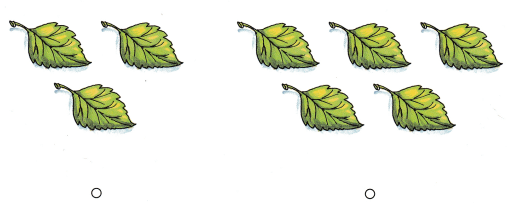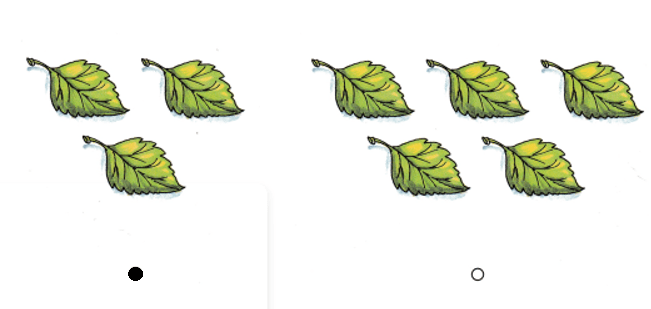# Texas Go Math Kindergarten Lesson 6.5 Answer Key Make a Set

Refer to our Texas Go Math Kindergarten Answer Key Pdf to score good marks in the exams. Test yourself by practicing the problems from Texas Go Math Kindergarten Lesson 6.5 Answer Key Make a Set.

## Texas Go Math Kindergarten Lesson 6.5 Answer Key Make a Set

Explore

DIRECTIONS: Make a set of 6 counters in the top workspace. Draw the counters. Trace the number. In the bottom workspace, make a set of counters that has two fewer than 6. Draw the counters. Write the number.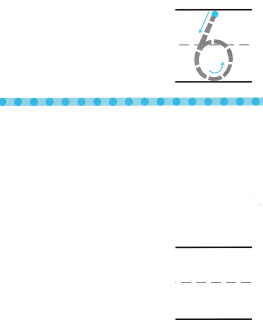Explanation:
Number of counters = 6.
2 Less than 6 = 6 – 2 = 4.

Share and Show

DIRECTIONS: 1. Use counters to make a set that is two more than 3. Draw the counters. Write the number. 2. Use counters to make a set that is the same as 3. Draw the counters. Write the number.

Question 1.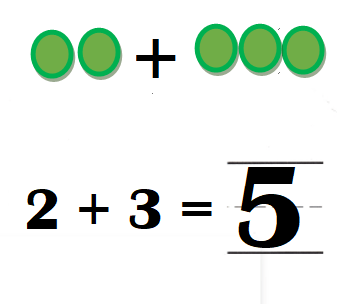Explanation:
Set that is two more than 3 = 2 + 3 = 5.

Question 2.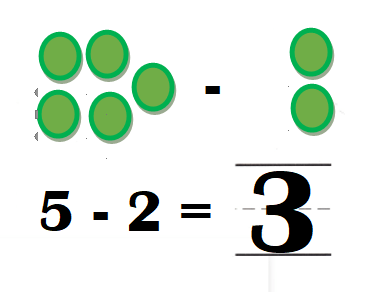Explanation:
Set that is the same as 3 = 5 – 2 = 3.

DIRECTIONS: 3. Use counters to make a set that is less than 7. Draw the counters. Write the number. 4. Use counters to make a set that is more than 4. Draw the counters. Write the number.

Question 3.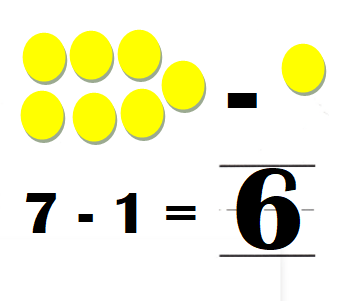Explanation:
Set that is less than 7 = 7 – 1 = 6.

Question 4.Explanation:
Set that is more than 4 = 4 + 1 = 5.

HOME ACTIVITY • Choose a number between 1 and 10. Using household items, have your child create a set that ¡s more than or less than that number.
He has created one less than the number 10.

Explanation:
I have given him 10 plastic spoons of different colors. He has counted them and later he hide a spoon and counted and told. There are only 9 spoons .

DIRECTIONS: 5. Morgan found two leaves. Joe found two more leaves than Morgan. How many leaves did Joe find? Draw Joe’s leaves. Write the number. 6. Choose the correct answer. Caleb has 6 beach balls. Which set has more beach balls than Caleb has?

Problem Solving
Question 5.Explanation:
Number of leaves Morgan found = 2.
Joe found two more leaves than Morgan.
=> Number of leaves Joe found = Number of leaves Morgan found + 2
= 2 + 2

Question 6.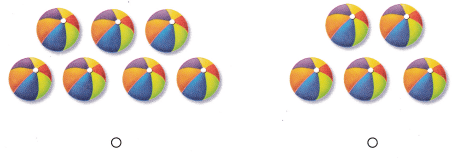Explanation:
Number of beach balls Caleb has = 6.
Set that has more beach balls than Caleb has = Number of beach balls Caleb has + 1
= 6 + 1
= 7.
5 < 6.
7 > 6.

### Texas Go Math Kindergarten Lesson 6.5 Homework and Practice Answer Key

DIRECTIONS: 1. Draw some counters to make a set that is less than 10. Write the number. 2. Draw some counters to make a set that is more than 6. Write the number.

Question 1.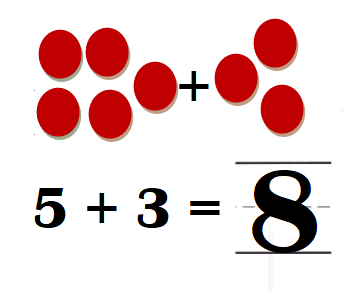Explanation:
Number of red counters = 5 + 3 = 8.
8 < 10.

Question 2.Explanation:
Number of red counters = 5 + 3 = 8.
8 > 6.

DIRECTIONS: Choose the correct answer. 3. Jose has 7 toy cars. Which set has more toy cars than Jose? 4. Marta has 4 leaves. Which set has a number of leaves that is less than 4?

Lesson Check
Question 3.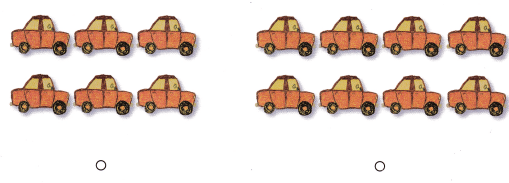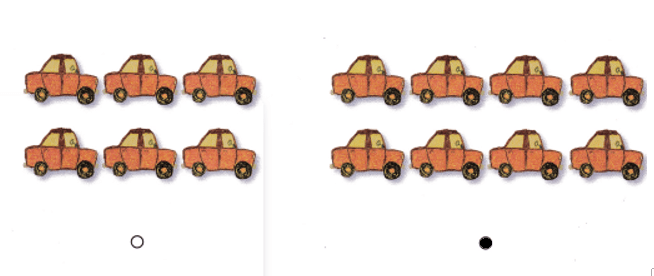Explanation:
Jose has 7 toy cars.
Set that has more toy cars than Jose = 7 + 1 = 8.
6 < 7.
8 > 7.

Question 4.